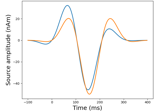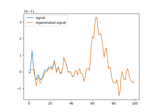# mne.time_frequency.fit_iir_model_raw¶

mne.time_frequency.fit_iir_model_raw(raw, order=2, picks=None, tmin=None, tmax=None, verbose=None)[source]

Fit an AR model to raw data and creates the corresponding IIR filter.

The computed filter is fitted to data from all of the picked channels, with frequency response given by the standard IIR formula:

$H(e^{jw}) = \frac{1}{a + ae^{-jw} + ... + a[n]e^{-jnw}}$
Parameters
rawRaw object

An instance of Raw.

orderint

Order of the FIR filter.

picks

Channels to include. Slices and lists of integers will be interpreted as channel indices. In lists, channel type strings (e.g., ['meg', 'eeg']) will pick channels of those types, channel name strings (e.g., ['MEG0111', 'MEG2623'] will pick the given channels. Can also be the string values “all” to pick all channels, or “data” to pick data channels. None (default) will pick good data channels. Note that channels in info['bads'] will be included if their names or indices are explicitly provided.

tminfloat

The beginning of time interval in seconds.

tmaxfloat

The end of time interval in seconds.

verbose

If not None, override default verbose level (see mne.verbose() and Logging documentation for more). If used, it should be passed as a keyword-argument only.

Returns
bndarray

Numerator filter coefficients.

andarray

Denominator filter coefficients.

## Examples using mne.time_frequency.fit_iir_model_raw¶